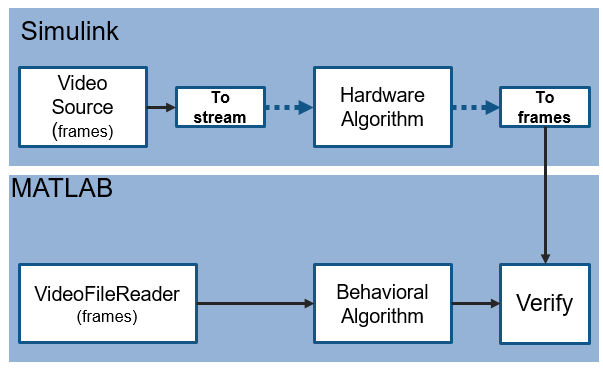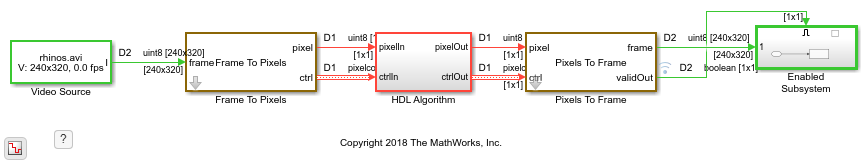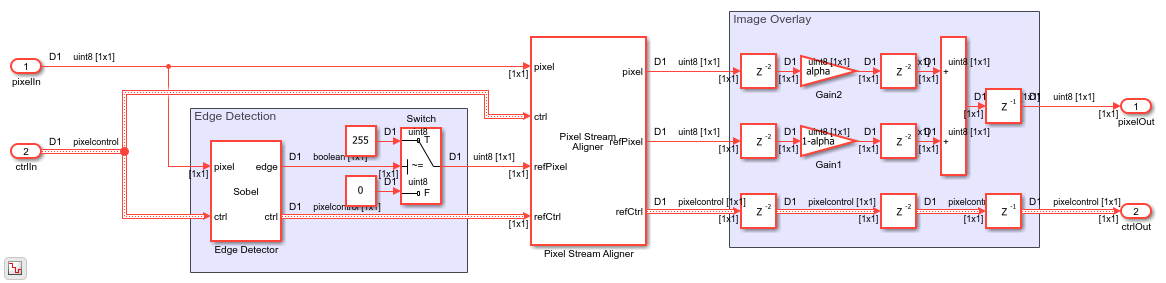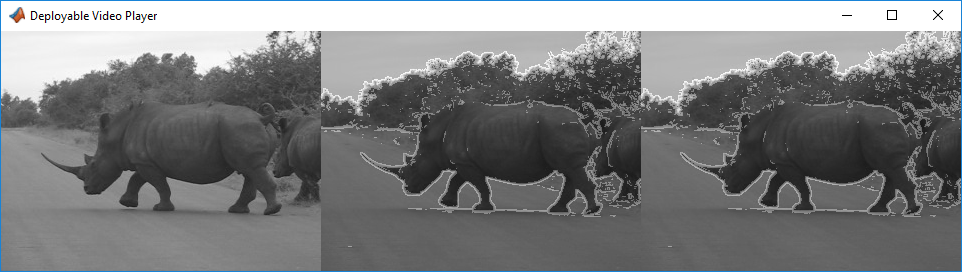# Convert MATLAB Vision Algorithm to Hardware-Targeted Simulink Model

This example shows how to create a hardware-targeted design in Simulink® that implements the same behavior as a MATLAB® reference design.

### Workflow

Image Processing Toolbox™ and Computer Vision Toolbox™ functions operate on framed, floating-point and integer data and provide excellent behavioral references. Hardware designs must use streaming Boolean or fixed-point data.

This example shows how to perform a framed image processing operation in MATLAB, and then implement the same operation in a Simulink model using streaming data. The Simulink model converts the input video to a pixel stream for hardware-friendly design. The same data is applied to both the hardware algorithm in Simulink and the behavioral algorithm in MATLAB. The Simulink model converts the output pixel stream to frames and exports those frames to MATLAB for comparison against the behavioral results.The MATLAB portion of this example loads the input video, runs the behavioral code, runs the Simulink model to import video frames and export modified video frames, and compares the MATLAB behavioral results with the Simulink output frames.

### Video Source

Create a video reader object to import a video file into the MATLAB workspace. The video source file is 240p format. Create a video player object to display the input frame, Simulink filtered frame, and MATLAB reference frame.

```videoIn = VideoReader('rhinos.avi'); numFrm = 10; % active frame dimensions actPixelsPerLine = 320; actLines = 240; % dimensions including blanking totalPixelsPerLine = 402; totalLines = 324; % viewer for results viewer = vision.DeployableVideoPlayer(... 'Size','Custom',... 'CustomSize',[3*actPixelsPerLine actLines]); ```

### Edge Detection and Overlay

Detect edges in the video frames, and then overlay those edges onto the original frame. The overlay computation uses an `alpha` value to mix the two pixel values. The Simulink model also uses the `edgeThreshold` and `alpha` parameters specified here.

The MATLAB `edge` function interprets the threshold as a double-precision value from 0 to 1. Therefore, express the threshold as a fraction of the range of the `uint8` data type, from 0 to 255. The pixel values returned by the `edge` function are `logical` data type. To convert these pixel values to `uint8` type for overlay, multiply by 255. This scaling operation converts logical ones to 255 and logical zeros stay 0.

```edgeThreshold = 8; alpha = 0.75; frmFull = uint8(zeros(actLines,actPixelsPerLine,numFrm)); frmRef = frmFull; for f = 1:numFrm frmFull(:,:,f) = rgb2gray(readFrame(videoIn)); edges = edge(frmFull(:,:,f),'Sobel',edgeThreshold/255,'nothinning'); edges8 = 255*uint8(edges)*(1-alpha); frmRef(:,:,f) = alpha*frmFull(:,:,f) + edges8; viewer([edges edges8 frmRef(:,:,f)]); end ```

### Set Up for Simulink Simulation

The Simulink model loads the input video into the model using a Video Source block. Configure the sample time of the model using the `totPixPerFrame` variable. This value includes the inactive pixel regions around the 240-by-320 frames. The Video Source sample time is 1 time step per frame, and the rate in the streaming pixel sections of the model is 1/ `totPixPerFrame` . Set the length of the simulation with the `simTime` variable.

```totPixPerFrame = totalPixelsPerLine*totalLines; simTime = (numFrm+1)*totPixPerFrame; modelname = 'VerifySLDesignAgainstMLReference'; open_system(modelname); set_param(modelname,'SampleTimeColors','on'); set_param(modelname,'SimulationCommand','Update'); set_param(modelname,'Open','on'); ```### Hardware-Targeted Algorithm

The HDL Algorithm subsystem is designed to support HDL code generation.

The subsystem uses the Edge Detector block to find edges. The output of the block is a stream of `boolean` pixel values. The model scales these values to `uint8` data type values for overlay.

The block returns the pixel stream of detected edges after several lines of latency, due to internal line buffers and filter logic. Before performing overlay, the model must delay the input stream to match the edge stream. The Pixel Stream Aligner block performs this alignment using the control signals of the output edge stream as a reference. This block stores the input stream in a FIFO until the detected edges are available.

The Image Overlay subsystem scales both streams by the `alpha` ratio and adds them together. With hardware implementation in mind, the Image Overlay subsystem includes pipeline stages around each multiplier and after the adder.

For more details of this edge detector design, see the Edge Detection and Image Overlay example.

```open_system([modelname '/HDL Algorithm']); ```Run the Simulink model to return ten frames overlaid with the detected edges.

```sim('VerifySLDesignAgainstMLReference'); ```

### Compare Simulink Results with MATLAB Results

Compare each video frame returned from Simulink with the result returned by the MATLAB behavioral code. The images look very similar but have small pixel value differences due to overlay mixing. The MATLAB overlay mixing is done using floating-point values, and the Simulink overlay mixing is done using fixed-point values. This comparison counts pixels in each frame whose values differ by more than 2 and calculates the peak-signal-to-noise ratio (PSNR) between the frames. To view the detailed differences at each frame, uncomment the last two lines in the loop.

``` for f = 1:numFrm frmResult = frmOut.signals.values(:,:,f); viewer([frmFull(:,:,f) frmResult frmRef(:,:,f)]); diff = frmRef(:,:,f) - frmResult; errcnt = sum(diff(:) > 2); noisecheck = psnr(frmRef(:,:,f),frmResult); fprintf(['\nFrame #%d has %d pixels that differ from behavioral result (by more than 2). PSNR = %2.2f\n'],f,errcnt,noisecheck); %bar3(diff); %viewer([frmResult frmRef(:,:,f) diff]); end ```
```Frame #1 has 2 pixels that differ from behavioral result (by more than 2). PSNR = 48.33 Frame #2 has 1 pixels that differ from behavioral result (by more than 2). PSNR = 48.72 Frame #3 has 1 pixels that differ from behavioral result (by more than 2). PSNR = 48.80 Frame #4 has 2 pixels that differ from behavioral result (by more than 2). PSNR = 48.66 Frame #5 has 2 pixels that differ from behavioral result (by more than 2). PSNR = 48.70 Frame #6 has 4 pixels that differ from behavioral result (by more than 2). PSNR = 48.27 Frame #7 has 2 pixels that differ from behavioral result (by more than 2). PSNR = 48.88 Frame #8 has 3 pixels that differ from behavioral result (by more than 2). PSNR = 48.58 Frame #9 has 3 pixels that differ from behavioral result (by more than 2). PSNR = 48.55 Frame #10 has 3 pixels that differ from behavioral result (by more than 2). PSNR = 48.53 ```### Generate HDL Code and Verify Its Behavior

Once your design is working in simulation, you can use HDL Coder™ to generate HDL code and a test bench for the HDL Algorithm subsystem.

```makehdl([modelname '/HDL Algorithm']) % Generate HDL code makehdltb([modelname '/HDL Algorithm']) % Generate HDL Test bench ```